Скачать презентацию MOLARITY MOLALITY DILUTIONS PERCENTS Molarity

02f6e8a9b201ee859db0f142667829e2.ppt

• Количество слайдов: 10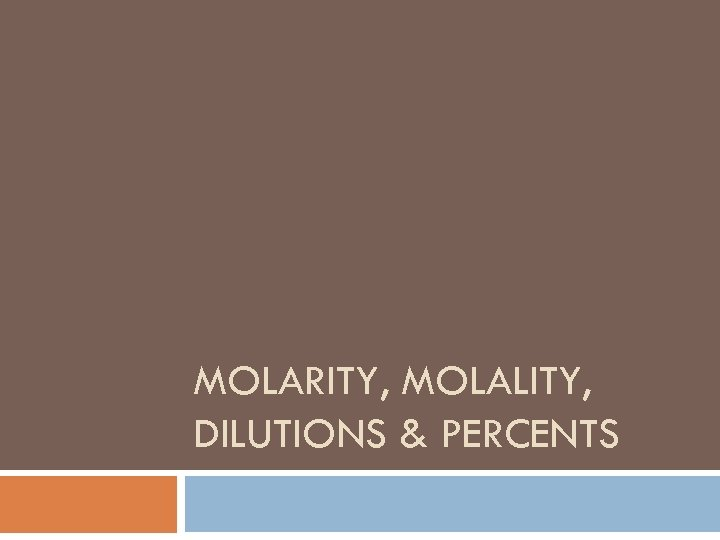MOLARITY, MOLALITY, DILUTIONS & PERCENTS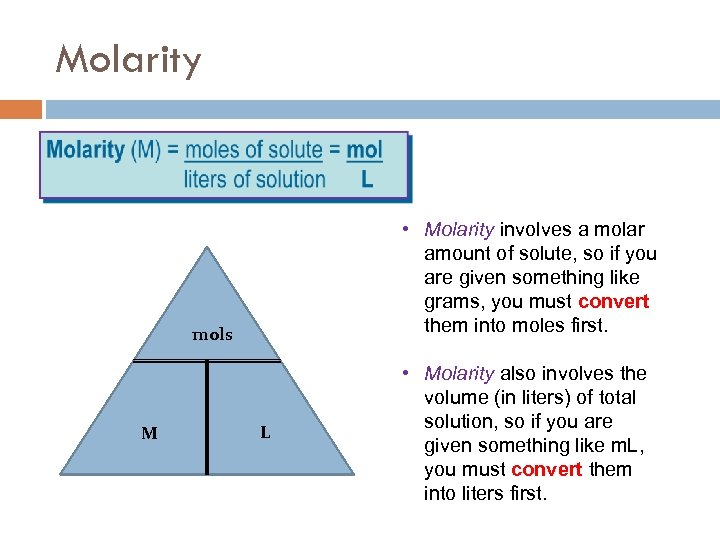Molarity • Molarity involves a molar amount of solute, so if you are given something like grams, you must convert them into moles first. mols M L • Molarity also involves the volume (in liters) of total solution, so if you are given something like m. L, you must convert them into liters first.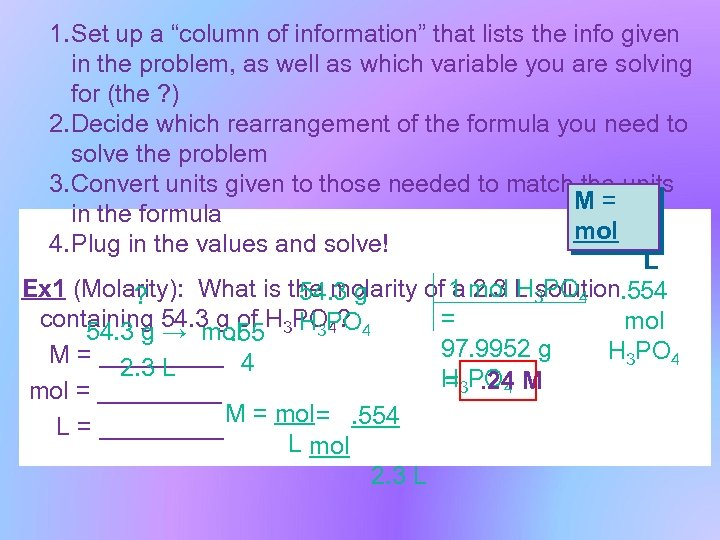1. Set up a “column of information” that lists the info given in the problem, as well as which variable you are solving for (the ? ) 2. Decide which rearrangement of the formula you need to solve the problem 3. Convert units given to those needed to match the units M= in the formula mol 4. Plug in the values and solve! L Ex 1 (Molarity): What is the molarity of 1 mol L 3 PO 4. 554 a 2. 3 H solution 54. 3 g ? = containing 54. 3 g of H 3 POPO 4 mol H 3 4? 54. 3 g → mol. 55 97. 9952 g H 3 PO 4 M = _____ 4 2. 3 L H 3 PO 4 M =. 24 mol = _____ M = mol =. 554 L = _____ L mol 2. 3 L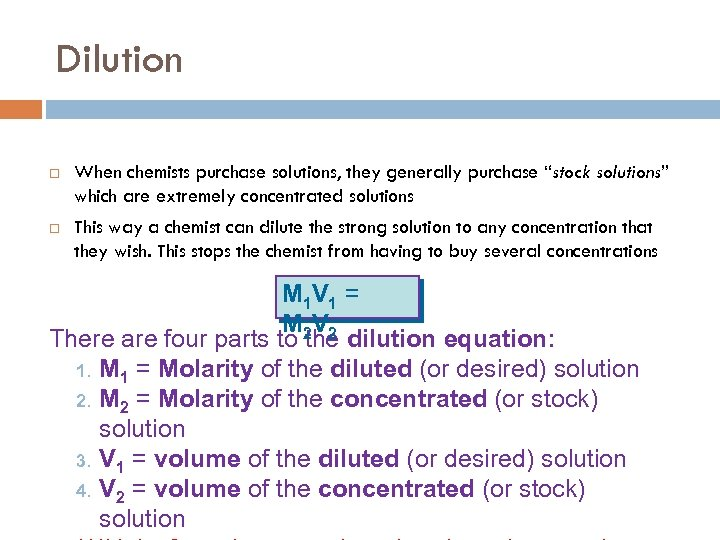Dilution When chemists purchase solutions, they generally purchase “stock solutions” which are extremely concentrated solutions This way a chemist can dilute the strong solution to any concentration that they wish. This stops the chemist from having to buy several concentrations M 1 V 1 = M 2 V 2 There are four parts to the dilution equation: 1. M 1 = Molarity of the diluted (or desired) solution 2. M 2 = Molarity of the concentrated (or stock) solution 3. V 1 = volume of the diluted (or desired) solution 4. V 2 = volume of the concentrated (or stock) solution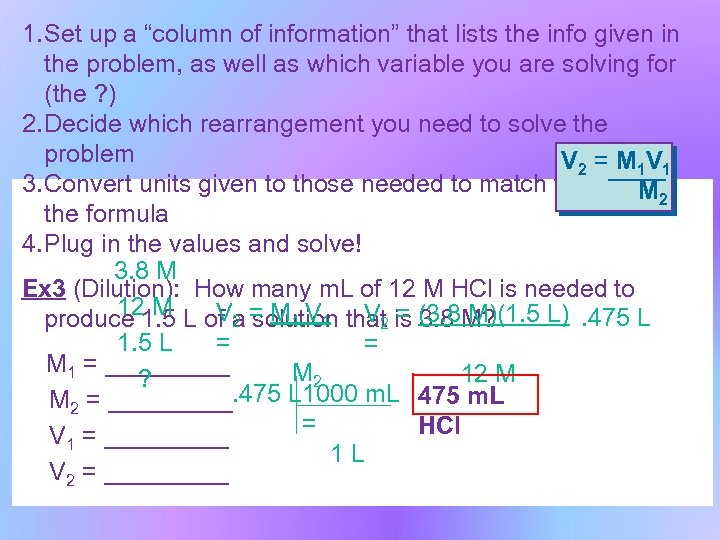1. Set up a “column of information” that lists the info given in the problem, as well as which variable you are solving for (the ? ) 2. Decide which rearrangement you need to solve the problem V 2 = M 1 V 1 3. Convert units given to those needed to match the units in M 2 the formula 4. Plug in the values and solve! 3. 8 M Ex 3 (Dilution): How many m. L of 12 M HCl is needed to 12 M V 2 solution V 2 = 3. 8 M)(1. 5 L). 475 L produce 1. 5 L of a = M 1 V 1 that is (3. 8 M? = 1. 5 L = M 1 = _____ M 2 12 M ? M 2 = _____. 475 L 1000 m. L 475 m. L = HCl V 1 = _____ 1 L V 2 = _____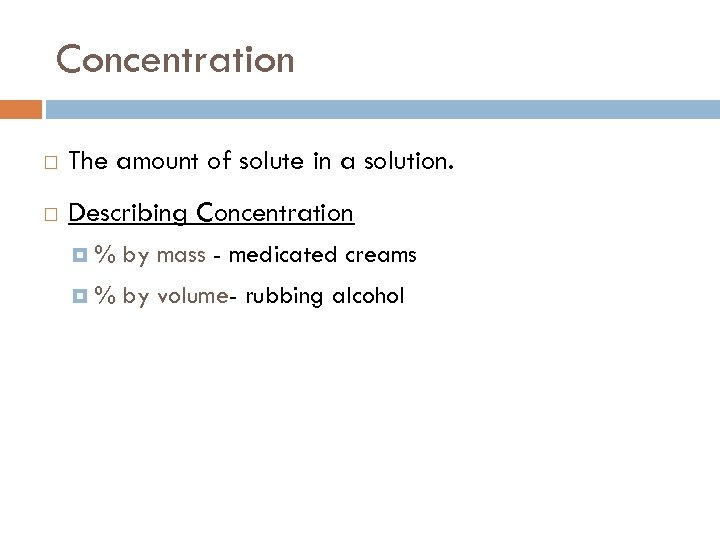Concentration The amount of solute in a solution. Describing Concentration % by mass - medicated creams % by volume- rubbing alcohol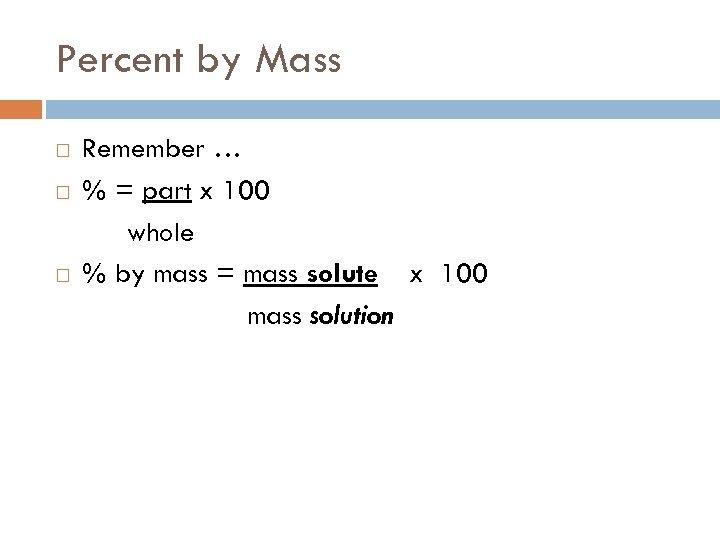Percent by Mass Remember … % = part x 100 whole % by mass = mass solute x 100 mass solution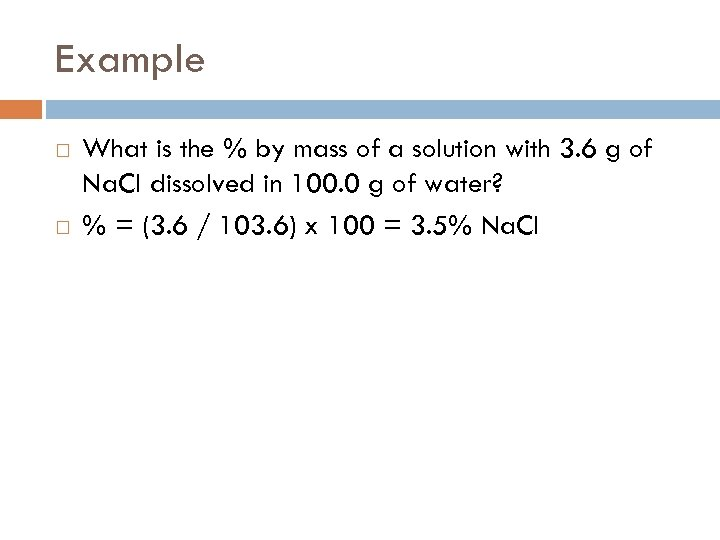Example What is the % by mass of a solution with 3. 6 g of Na. Cl dissolved in 100. 0 g of water? % = (3. 6 / 103. 6) x 100 = 3. 5% Na. Cl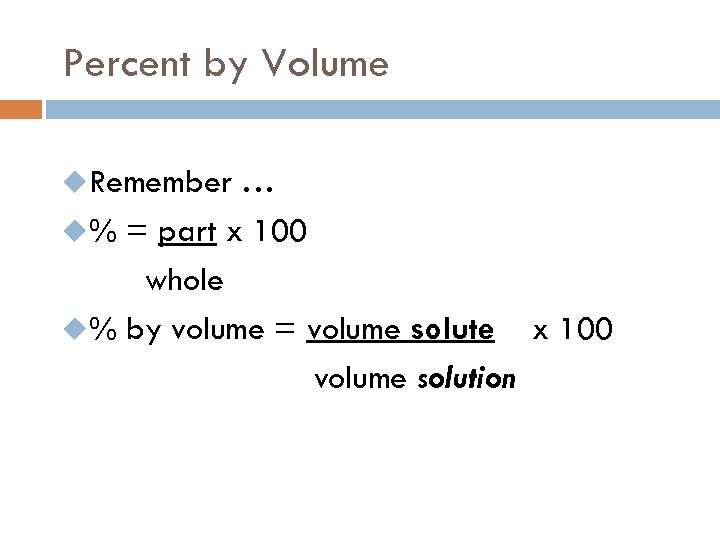Percent by Volume u. Remember … u% = part x 100 whole u% by volume = volume solute x 100 volume solution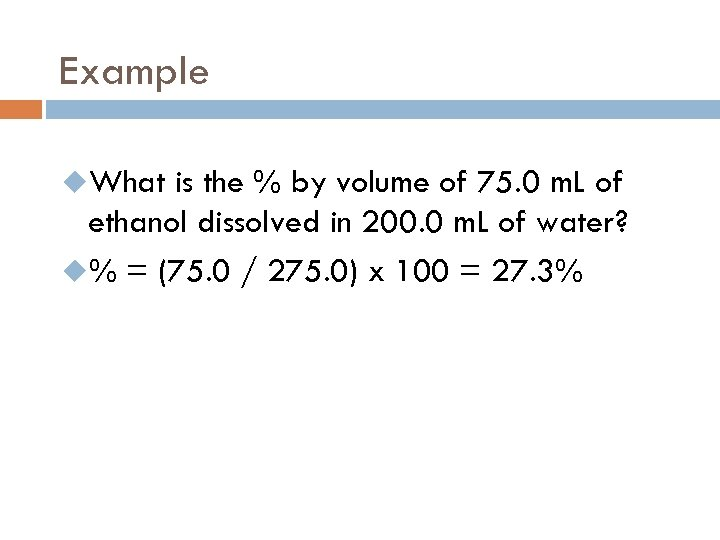Example u. What is the % by volume of 75. 0 m. L of ethanol dissolved in 200. 0 m. L of water? u% = (75. 0 / 275. 0) x 100 = 27. 3%